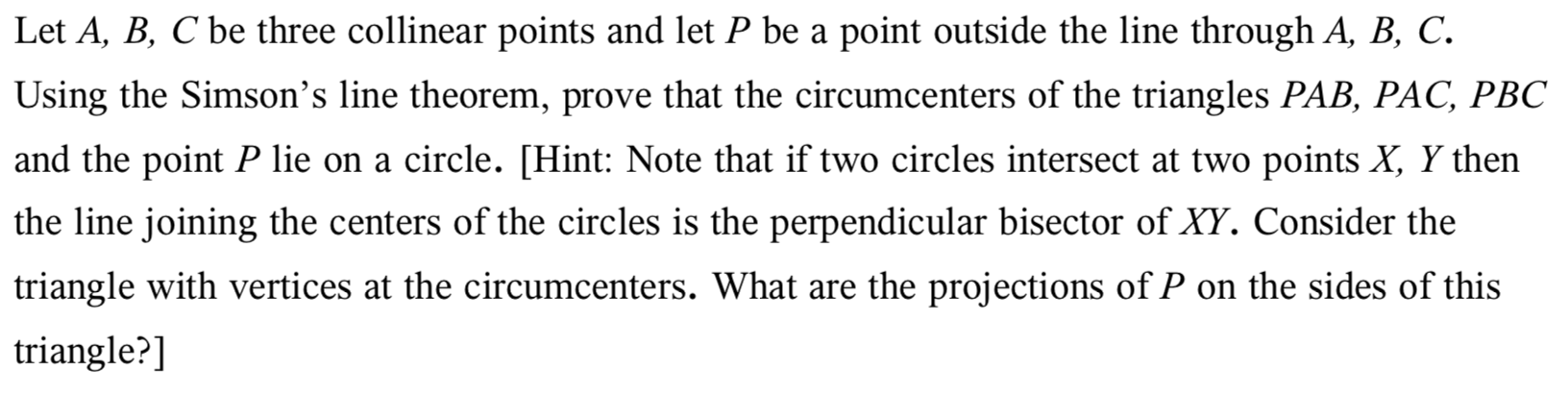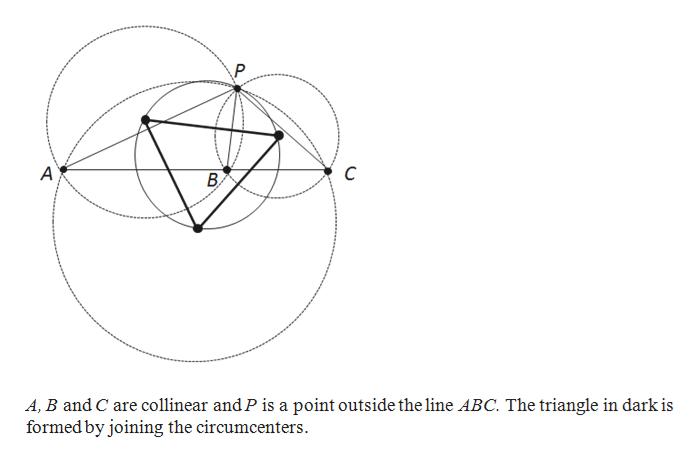# Let A, B, C be three collinear points and let P be a point outside the line through A, B, C.Using the Simson's line theorem, prove that the circumcenters of the triangles PAB, PAC, PBCand the point P lie on a circle. [Hint: Note that if two circles intersect at two points X, Y thenthe line joining the centers of the circles is the perpendicular bisector of XY. Consider thetriangle with vertices at the circumcenters. What are the projections ofP on the sides of thistriangle?]

Question
60 viewshelp_outlineImage TranscriptioncloseLet A, B, C be three collinear points and let P be a point outside the line through A, B, C. Using the Simson's line theorem, prove that the circumcenters of the triangles PAB, PAC, PBC and the point P lie on a circle. [Hint: Note that if two circles intersect at two points X, Y then the line joining the centers of the circles is the perpendicular bisector of XY. Consider the triangle with vertices at the circumcenters. What are the projections ofP on the sides of this triangle?] fullscreen
check_circle

Step 1

Sketch the diagrams with the help of geometrical ...help_outlineImage TranscriptioncloseC A A, B and C are collinear and P is a point outside the line ABC. The triangle in dark is formed by joining the circumcenters fullscreen

### Want to see the full answer?

See Solution

#### Want to see this answer and more?

Solutions are written by subject experts who are available 24/7. Questions are typically answered within 1 hour.*

See Solution
*Response times may vary by subject and question.
Tagged in

### Math